edit

# Grove Thermal Imaging Camera IR Array MLX90641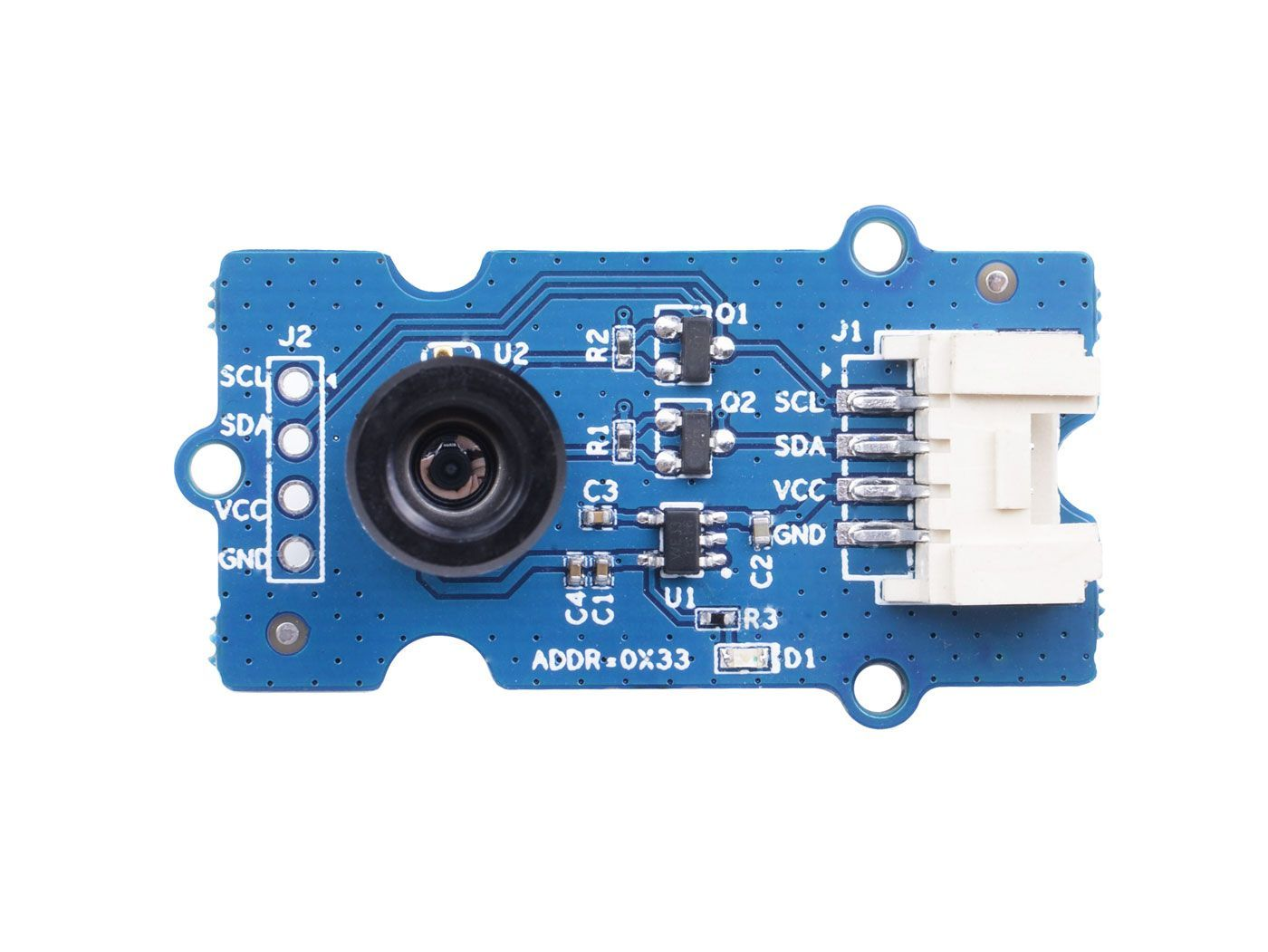This IR thermal camera carries a 16x12 array of thermal sensors (MLX90641) and it can detect the temperature of objects from far away with a center area accuracy of ±1℃ and average accuracy of ±1.5℃. In order to obtain the thermal images easily, the I2C protocol is used to get the low-resolution images from the camera. The FOV (Field of View) of this camera is 110°x75°, and the temperature measurement range is -40℃ to 300℃. In order to obtain the thermal image easily, I2C protocol is used to get the low-resolution image from the camera.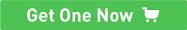Grove - Thermal Imaging Camera / IR Array MLX90640 110 degree## Features¶

• Compact size 16x12 pixel IR thermal sensor array (MLX90641)
• High FOV (field-of-view) of 110°x75° to capture more area
• Wide temperature measurement range (-40℃~300℃)
• I2C Grove interface for easy communication with an MCU
• Fully calibrated IR array for convenient setup

## Specification¶

Item Grove - Thermal Imaging Camera - MLX90640 Grove - Thermal Imaging Camera - MLX90641
Thermal sensor 32X24 array MLX90640 16x12 array MLX90641
Operating Voltage 3V - 6V 3.3V - 5V
Current consumption ~18mA ~18mA
FOV(Field of View) 110°x75° 110°x75°
Temperature Measurement Range -40°C - 300°C -40°C - 300°C
Temperature Resolution ± 1.5°C ± 1.5°C (±1℃ at center area)
Refresh Rate 0.5Hz - 64Hz 0.5Hz - 64Hz
Interface I2C Grove interface I2C Grove interface

## Platforms Supported¶

Arduino Raspberry Pi BeagleBone Wio LinkIt ONE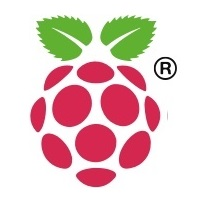## Getting Started¶

### Getting Started by Wio Terminal¶

Materials required

Wio Terminal Grove - Thermal Imaging Camera / IR Array MLX90641 110 degree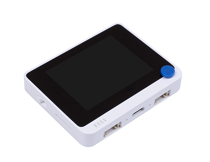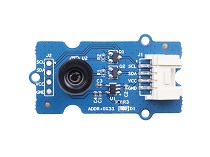Get one now Get one now

#### Hardware Connection¶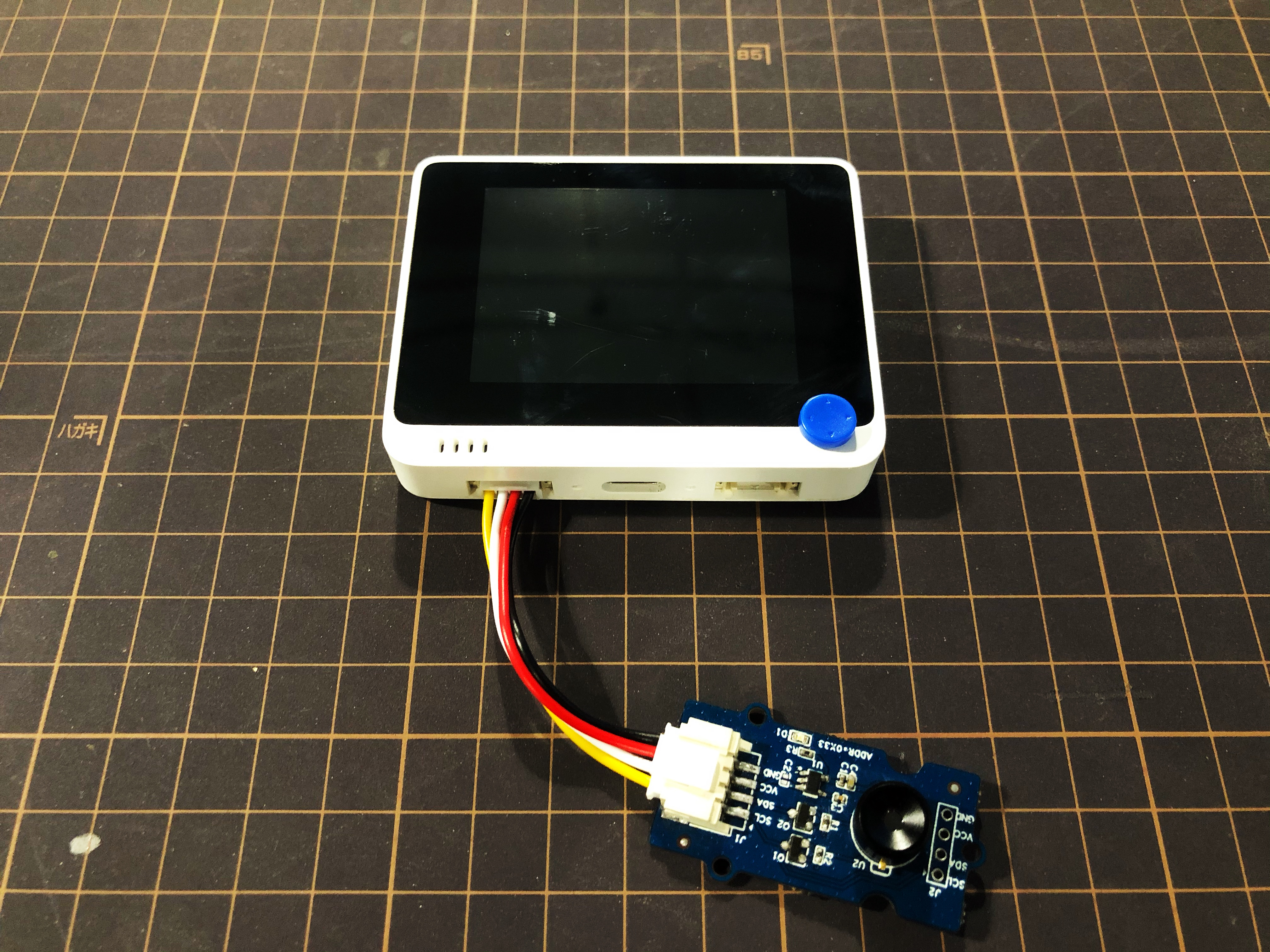Step 1. Plug Grove - Thermal Imaging Camera to Wio Terminal via Grove cable and also connect Wio Terminal to PC through a USB cable.

Note

If it is your first time playing Wio Terminal and not sure which interface to plug on Wio Terminal, please refer to Get Started with Wio Terminal.

Step 2. Copy the demo code into your Arduino IDE and upload.

Software

#include <Wire.h>
#include "MLX90641_API.h"
#include "MLX9064X_I2C_Driver.h"
#include <TFT_eSPI.h>                // Include the graphics library (this includes the sprite functions)

const byte MLX90641_address = 0x33; //Default 7-bit unshifted address of the MLX90641
#define TA_SHIFT 12 //Default shift for MLX90641 in open air
#define debug  Serial
uint16_t eeMLX90641;
float MLX90641To;
uint16_t MLX90641Frame;
paramsMLX90641 MLX90641;
int errorno = 0;

TFT_eSPI    tft = TFT_eSPI();
TFT_eSprite Display = TFT_eSprite(&tft);  // Create Sprite object "img" with pointer to "tft" object
// the pointer is used by pushSprite() to push it onto the TFT

unsigned long CurTime;

uint16_t TheColor;
uint16_t MinTemp = 25;
uint16_t MaxTemp = 38;

// variables for interpolated colors
byte red, green, blue;

// variables for row/column interpolation
byte i, j, k, row, col, incr;
float intPoint, val, a, b, c, d, ii;
byte aLow, aHigh;

// size of a display "pixel"
byte BoxWidth = 3;
byte BoxHeight = 3;

int x, y;
char buf;

// variable to toggle the display grid
int ShowGrid = -1;

// array for the interpolated array
float HDTemp;

void setup() {
Wire.begin();
Wire.setClock(2000000); //Increase I2C clock speed to 2M
debug.begin(115200); //Fast debug as possible

// start the display and set the background to black

if (isConnected() == false) {
while (1);
}
//Get device parameters - We only have to do this once
int status;
errorno = status;//MLX90641_CheckEEPROMValid(eeMLX90641);//eeMLX90641 & 0x0040;//

if (status != 0) {
while(1);
}

status = MLX90641_ExtractParameters(eeMLX90641, &MLX90641);
//errorno = status;
if (status != 0) {
debug.println("Parameter extraction failed");
while(1);
}

//Once params are extracted, we can release eeMLX90641 array

MLX90641_SetRefreshRate(MLX90641_address, 0x05); //Set rate to 16Hz

tft.begin();
tft.setRotation(3);
tft.fillScreen(TFT_BLACK);
Display.createSprite(TFT_HEIGHT, TFT_WIDTH);
Display.fillSprite(TFT_BLACK);

// get the cutoff points for the color interpolation routines
// note this function called when the temp scale is changed
Getabcd();

// draw a legend with the scale that matches the sensors max and min
DrawLegend();
}
void loop() {
// draw a large white border for the temperature area
Display.fillRect(10, 10, 220, 220, TFT_WHITE);
for (byte x = 0 ; x < 2 ; x++) {

float vdd = MLX90641_GetVdd(MLX90641Frame, &MLX90641);
float Ta = MLX90641_GetTa(MLX90641Frame, &MLX90641);

float tr = Ta - TA_SHIFT; //Reflected temperature based on the sensor ambient temperature
float emissivity = 0.95;

MLX90641_CalculateTo(MLX90641Frame, &MLX90641, emissivity, tr, MLX90641To);
}

interpolate_image(MLX90641To,12,16,HDTemp,80,80);

//display the 80 x 80 array

//Crosshair in the middle of the screen
Display.drawCircle(115, 115, 5, TFT_WHITE);
Display.drawFastVLine(115, 105, 20, TFT_WHITE);
Display.drawFastHLine(105, 115, 20, TFT_WHITE);
//Displaying the temp at the middle of the Screen

//Push the Sprite to the screen
Display.pushSprite(0, 0);

tft.setRotation(3);
tft.setTextColor(TFT_WHITE);
tft.drawFloat(HDTemp[35 * 80 + 35], 2, 90, 20);

}
//Returns true if the MLX90640 is detected on the I2C bus
boolean isConnected() {
if (Wire.endTransmission() != 0) {
return (false);    //Sensor did not ACK
}
return (true);
}
// function to display the results

tft.setRotation(4);

// rip through 70 rows
for (row = 0; row < 70; row ++) {

// fast way to draw a non-flicker grid--just make every 10 MLX90641To 2x2 as opposed to 3x3
// drawing lines after the grid will just flicker too much
if (ShowGrid < 0) {
BoxWidth = 3;
}
else {
if ((row % 10 == 9) ) {
BoxWidth = 2;
}
else {
BoxWidth = 3;
}
}
// then rip through each 70 cols
for (col = 0; col < 70; col++) {

// fast way to draw a non-flicker grid--just make every 10 MLX90641To 2x2 as opposed to 3x3
if (ShowGrid < 0) {
BoxHeight = 3;
}
else {
if ( (col % 10 == 9)) {
BoxHeight = 2;
}
else {
BoxHeight = 3;
}
}
// finally we can draw each the 70 x 70 points, note the call to get interpolated color
Display.fillRect((row * 3) + 15, (col * 3) + 15, BoxWidth, BoxHeight, GetColor(HDTemp[row * 80 + col]));
}
}

}
// my fast yet effective color interpolation routine
uint16_t GetColor(float val) {

/*
pass in value and figure out R G B
several published ways to do this I basically graphed R G B and developed simple linear equations
again a 5-6-5 color display will not need accurate temp to R G B color calculation

equations based on

*/

red = constrain(255.0 / (c - b) * val - ((b * 255.0) / (c - b)), 0, 255);

if ((val > MinTemp) & (val < a)) {
green = constrain(255.0 / (a - MinTemp) * val - (255.0 * MinTemp) / (a - MinTemp), 0, 255);
}
else if ((val >= a) & (val <= c)) {
green = 255;
}
else if (val > c) {
green = constrain(255.0 / (c - d) * val - (d * 255.0) / (c - d), 0, 255);
}
else if ((val > d) | (val < a)) {
green = 0;
}

if (val <= b) {
blue = constrain(255.0 / (a - b) * val - (255.0 * b) / (a - b), 0, 255);
}
else if ((val > b) & (val <= d)) {
blue = 0;
}
else if (val > d) {
blue = constrain(240.0 / (MaxTemp - d) * val - (d * 240.0) / (MaxTemp - d), 0, 240);
}

// use the displays color mapping function to get 5-6-5 color palet (R=5 bits, G=6 bits, B-5 bits)
return Display.color565(red, green, blue);

}

// function to get the cutoff points in the temp vs RGB graph
void Getabcd() {

a = MinTemp + (MaxTemp - MinTemp) * 0.2121;
b = MinTemp + (MaxTemp - MinTemp) * 0.3182;
c = MinTemp + (MaxTemp - MinTemp) * 0.4242;
d = MinTemp + (MaxTemp - MinTemp) * 0.8182;

}
float get_point(float *p, uint8_t rows, uint8_t cols, int8_t x, int8_t y)
{
if (x < 0)
{
x = 0;
}
if (y < 0)
{
y = 0;
}
if (x >= cols)
{
x = cols - 1;
}
if (y >= rows)
{
y = rows - 1;
}
return p[y * cols + x];
}

void set_point(float *p, uint8_t rows, uint8_t cols, int8_t x, int8_t y, float f)
{
if ((x < 0) || (x >= cols))
{
return;
}
if ((y < 0) || (y >= rows))
{
return;
}
p[y * cols + x] = f;
}

// src is a grid src_rows * src_cols
// dest is a pre-allocated grid, dest_rows*dest_cols
void interpolate_image(float *src, uint8_t src_rows, uint8_t src_cols,
float *dest, uint8_t dest_rows, uint8_t dest_cols)
{
float mu_x = (src_cols - 1.0) / (dest_cols - 1.0);
float mu_y = (src_rows - 1.0) / (dest_rows - 1.0);

for (uint8_t y_idx = 0; y_idx < dest_rows; y_idx++)
{
for (uint8_t x_idx = 0; x_idx < dest_cols; x_idx++)
{
float x = x_idx * mu_x;
float y = y_idx * mu_y;

float frac_x = x - (int)x; // we only need the ~delta~ between the points
float frac_y = y - (int)y; // we only need the ~delta~ between the points
float out = bicubicInterpolate(adj_2d, frac_x, frac_y);
set_point(dest, dest_rows, dest_cols, x_idx, y_idx, out);
}
}
}

// p is a list of 4 points, 2 to the left, 2 to the right
float cubicInterpolate(float p[], float x)
{
float r = p + (0.5 * x * (p - p + x * (2.0 * p - 5.0 * p + 4.0 * p - p + x * (3.0 * (p - p) + p - p))));
return r;
}

// p is a 16-point 4x4 array of the 2 rows & columns left/right/above/below
float bicubicInterpolate(float p[], float x, float y)
{
float arr = {0, 0, 0, 0};
arr = cubicInterpolate(p + 0, x);
arr = cubicInterpolate(p + 4, x);
arr = cubicInterpolate(p + 8, x);
arr = cubicInterpolate(p + 12, x);
return cubicInterpolate(arr, y);
}

// src is rows*cols and dest is a 4-point array passed in already allocated!
void get_adjacents_1d(float *src, float *dest, uint8_t rows, uint8_t cols, int8_t x, int8_t y)
{
// pick two items to the left
dest = get_point(src, rows, cols, x - 1, y);
dest = get_point(src, rows, cols, x, y);
// pick two items to the right
dest = get_point(src, rows, cols, x + 1, y);
dest = get_point(src, rows, cols, x + 2, y);
}

// src is rows*cols and dest is a 16-point array passed in already allocated!
void get_adjacents_2d(float *src, float *dest, uint8_t rows, uint8_t cols, int8_t x, int8_t y)
{
float arr;
for (int8_t delta_y = -1; delta_y < 3; delta_y++)
{                                          // -1, 0, 1, 2
float *row = dest + 4 * (delta_y + 1); // index into each chunk of 4
for (int8_t delta_x = -1; delta_x < 3; delta_x++)
{ // -1, 0, 1, 2
row[delta_x + 1] = get_point(src, rows, cols, x + delta_x, y + delta_y);
}
}
}

// function to draw a legend
void DrawLegend() {

//color legend with max and min text
j = 0;

float inc = (MaxTemp - MinTemp ) / 160.0;

for (ii = MinTemp; ii < MaxTemp; ii += inc) {
tft.drawFastHLine(260, 200 - j++, 30, GetColor(ii));
}

tft.setTextSize(2);
tft.setCursor(245, 20);
tft.setTextColor(TFT_WHITE, TFT_BLACK);
sprintf(buf, "%2d/%2d", MaxTemp, (int) (MaxTemp * 1.12) + 32);
tft.print(buf);

tft.setTextSize(2);
tft.setCursor(245, 210);
tft.setTextColor(TFT_WHITE, TFT_BLACK);
sprintf(buf, "%2d/%2d", MinTemp, (int) (MinTemp * 1.12) + 32);
tft.print(buf);

}


Success

The outcome will display on the screen of Wio Terminal if everything goes well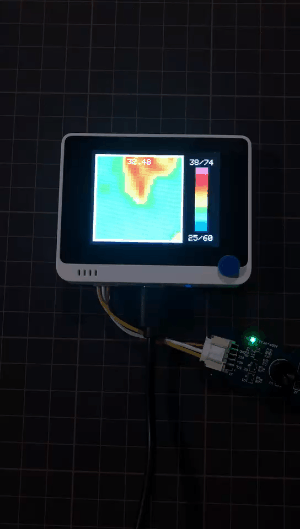## Tech Support¶

Please submit any technical issue into our forum.